Courses

# Study Notes for Gear Trains Mechanical Engineering Notes | EduRev

## Mechanical Engineering : Study Notes for Gear Trains Mechanical Engineering Notes | EduRev

The document Study Notes for Gear Trains Mechanical Engineering Notes | EduRev is a part of the Mechanical Engineering Course Theory of Machines (TOM).
All you need of Mechanical Engineering at this link: Mechanical Engineering

What is Gear Train?

• When two or more gears are made to mesh with each other to transmit power from one shaft to another, the combination is called gear trains.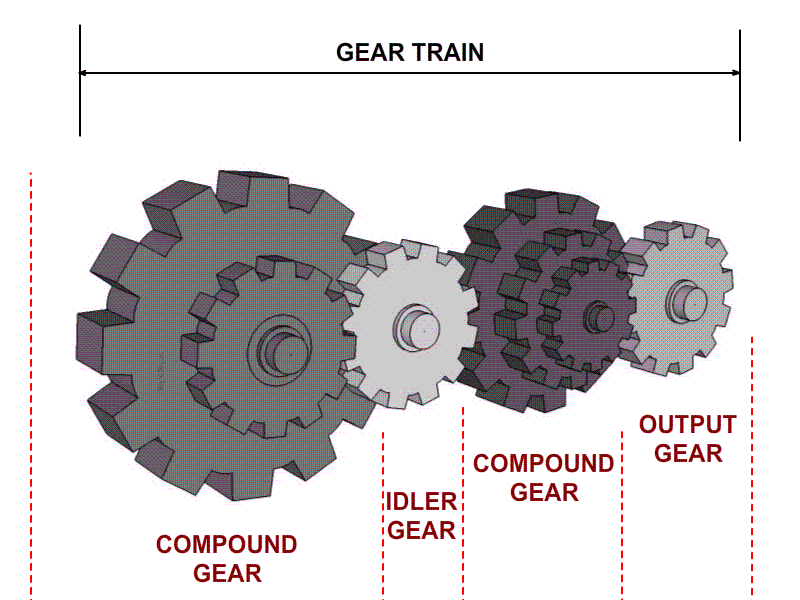• A gear train is a combination of gears used to transmit motion from one shaft to another. Gear trains are used to speed up or stepped down the speed of driven shaft.

Types of Gear Trains

1. Simple Gear Train: Series of gears, capable of receiving and transmitting motion from one gear to another is called a simple gear train.
Train value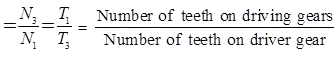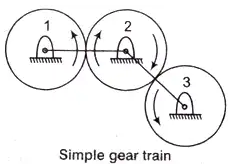Speed ratio = 1/Train value
The intermediate gears have no effect on the speed ratio and therefore they are known as idlers.
Try yourself:In a simple gear train, if the number of idle gears is odd, then the motion of driven gear will

2. Compound Gear Train: When a series of gears are connected in such a way that two or more gears rotate about an axis with the same angular velocity.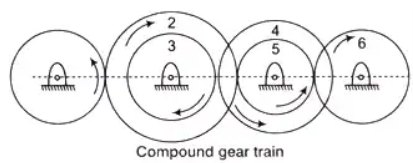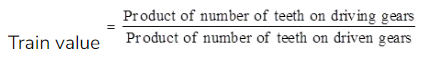3. Reverted Gear Train: If the axis of the first and last wheel of a compound gear coincide, it is called as reverted gear train.
Train valve,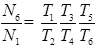If r is the pitch circle radius of a gear
r1 + r2 = r3 + r4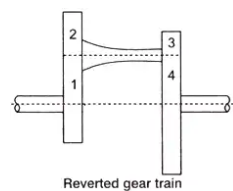4. Planetary or Epicyclic Gear Train: A gear train having a relative motion of axes is called a planetary or an epicyclic gear train. In an epicyclic train, the axis of at least one of the gears also moves relative to the frame. If the arm a is fixed the wheels S and P constitute a simple train. However if the wheel S is fixed so that arm a can rotate about the axis of S. The P would be moved around S therefore it is an epicyclic train.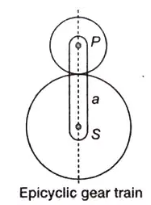5. In general, gear trains have 2 degree of freedom.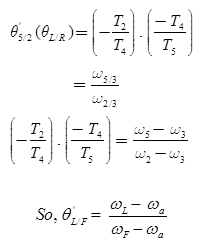First order kinematic coefficient

6. Torque in epicyclic Trains: Let NS, Na, Np, and NA be the speeds and TS, Ta, Tp, and TA the external transmitted by S, a, P and A
∑T = 0
TS + Ta + Tp + TA = 0
Since planet P can rotate on its our pin fixed to a but is not connected to anything outside.
Tp = 0
TS + Ta + TA = 0
Assuming no loss in power transistor
∑Tω = 0
TSNS + TaNa + TANA = 0
If efficiency η to deliver power to S.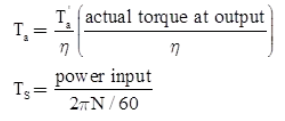7. Tabular Method: In tabular method, we apply following procedure. Assume gear makes m revolution and arm makes n revolution.
Consider clockwise rotation is positive and anticlockwise rotation is negative.

Analysis of Tabular Method: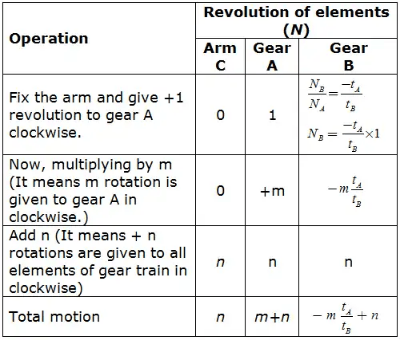On the basis of given condition, we can determine the values m and n. For example, if gear A is at rust, then
NA = 0
∴ m + n = 0  ....(i)
and arm is given +k revolution, then
n = k …(ii)
By solving Equations: (i) and (ii), we can find the values of m and n. Once we find out m and n then number of revolutions and direction of gear B can be determined as: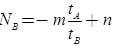Try yourself:When the axes of first and last gear are co-axial, then gear train is known as

Offer running on EduRev: Apply code STAYHOME200 to get INR 200 off on our premium plan EduRev Infinity!

## Theory of Machines (TOM)

94 videos|41 docs|28 tests

,

,

,

,

,

,

,

,

,

,

,

,

,

,

,

,

,

,

,

,

,

;